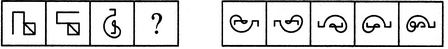# Non Verbal Reasoning - Analogy - Discussion

Discussion Forum : Analogy - Section 1 (Q.No. 19)
Directions to Solve

Each of the following questions consists of two sets of figures. Figures A, B, C and D constitute the Problem Set while figures 1, 2, 3, 4 and 5 constitute the Answer Set. There is a definite relationship between figures A and B. Establish a similar relationship between figures C and D by selecting a suitable figure from the Answer Set that would replace the question mark (?) in fig. (D).

19.

Select a suitable figure from the Answer Figures that would replace the question mark (?).(A)     (B)      (C)     (D)                  (1)      (2)      (3)      (4)      (5)
1
2
3
4
5
Explanation:
The figure rotates 90oACW and gets vertically inverted.
Discussion:
3 comments Page 1 of 1.

Gharti said:   12 months ago
I think the right answer is 2.
(1)

Anomie said:   1 year ago
Yeah, it should be laterally inverted, not vertically.

Manish said:   3 years ago
I think after moving 90 acw it gets horizontal inverted, not vertical inverted.

Am I right?